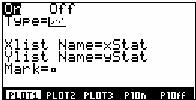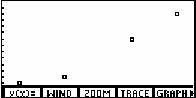# Knowledge Base

## Solution 10439: Plotting Points Using the TI-86.

### How do I plot a set of points using the TI-86?

The following example will demonstrate how to plot a set of points using the TI-86:

Data for this example:
xStat: {3,5,8,10}
yStat: {7,8,14,18}

Enter the data into the TI-86:
• Enter STAT mode by pressing [2nd][STAT].
• Press [F2] to select Edit.
• Enter the data in the xStat and yStat lists. (Note: to save data entry time, a frequency list, fStat, can be used. For this example, the frequency of each answer is 1, so clear the fStat list and leave it blank.)
• Press [2nd] [QUIT] to leave the editor.

Plot the coordinates:
• Press [2nd] [STAT] [F3] [F1].
• Turn the plot on by pressing [ENTER] then press the down arrow.
• Select [F1] to view a scatter plot.• Press [GRAPH] [F3] [MORE] [F5].